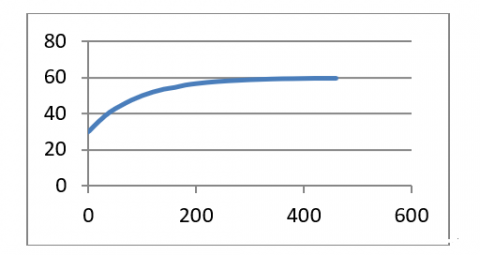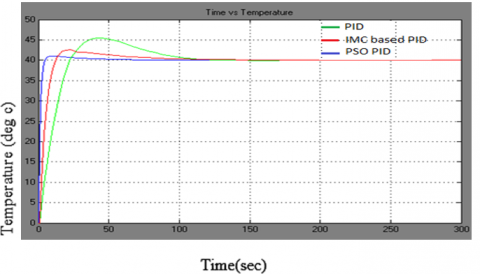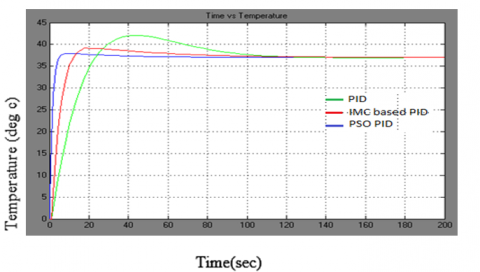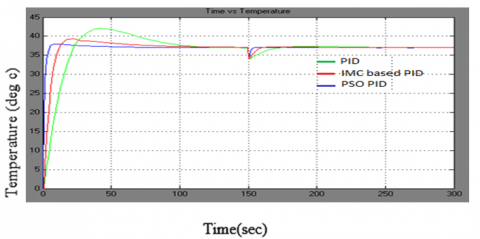# Design and Implementation of Internal Model Control and Particle Swarm Optimization Based PID for Heat Exchanger System

Design and Implementation of Internal Model Control and Particle Swarm Optimization Based PID for Heat Exchanger System

E. KesavanN. Gowthaman S. Tharani S. Manoharan E. Arunkumar

Karpagam College of Engineering, Coimbatore, India

Corresponding Author Email:
kesavanethiraj2013@gmail.com
Page:
386-390
|
DOI:
https://doi.org/10.18280/ijht.340306
|
Accepted:
|
Published:
30 September 2016
| Citation

OPEN ACCESS

Abstract:

Heat exchanger plays vital role in chemical plants because they can withstand wide range of pressure and temperature. The heat exchanger transmit heat from hot liquid to cold liquid in order to avoid the loss of heat in chemical processes. Here we proposed different tuning strategies of PID controller for control the cold water temperature at desired set point. The best controller tuning method is found out from the transient response performance and the error criteria. The simulation is performed using MATLAB for different tuning strategies of the PID controller. The model result ensure that the Particle Swarm Optimization based PID provides efficient control action than conventional PID and Internal model control based PID controller.

Keywords:

Heat exchanger system, PSO based PID controller, Cold water temperature.

1. Introduction

Heat exchangers  are widely used in gas industries, petro chemical industries and thermal power plants. The advantages of these heat exchangers are their high efficiency compact structure and low cost . Design of controller for heat exchanger is very challenging process due the aspects like measurement noise, uncertainty and the robustness of the system. The nonlinear system control can be classified in two types are tight control action and the smooth control action. Tight control action gives a faster control action than smooth control action . The PID controller is the best controller for controlling the heat exchanger due to the characteristics and structure of this controller. There are different tuning methods are available for tuning the controller parameters like controller gain, integral time and the derivative time . The widely Ziegler-Nichols method is not suitable for some kind of process dynamics . The heat exchangers important dynamics characteristic is dead time. The other PID tuning rules make the controllers effective for the different dynamics process like heat exchanger. Padhee has highlighted the limitation of feedback and feedback plus feed forward controller for the heat exchanger system . The determination of controller gains of the PID controller may not be perfect in terms of optimal control action. Other researchers have highlighted the evolutionary algorithms and optimization algorithms for tuning of PID controllers . This manuscript recommends particle swarm optimization based PID controller for heat exchanger systems.

2. Heat Exchanger System

The Shell and tube heat exchanger is widely used in chemical process industries. This type of heat exchanger is mainly consisting of a shell and many tubes. The heat exchanger system is used transfer the heat from hot water to cold water. The figure 1 shows the Structure of the heat exchanger system.

## figure_1.pngFigure 1. Heat exchanger setup

Tti-Tube inlet temperature.

Tto-Tube outlet temperature.

Fs-Shell inlet flow rate.

Ft-tube inlet flow rate.

Pt-Tube inlet pump.

Ps-Shell inlet pump

V1,V2,V3,V4,V5 and V6-Valves

The tube inlet pump connects the cold water reservoir to the tube inlet and the shell inlet pump connects the hot water reservoir to the shell inlet. The cold water flows through the tube and the hot water flows through the shell of the heat exchanger. The heat transfer rate between the shell and tube depends upon the flow rate of hot and cold water. The tube out let temperature is controlled by regulating the shell inlet flow rate. The shell inlet pump gets command signal from the controller  and regulates the flow rate depending upon the tube out let temperature.

3. Mathematical Model

The proper mathematical model is obtained from real time system by applying the step input to the process. The majority of the non linear process can be a first order plus dead time model. The total heat of the process can be represented by equation 1 .

$\mathrm{Q}_{\mathrm{f}}=\mathrm{Q}_{\mathrm{s}}+\sum_{\mathrm{i}=1}^{\mathrm{n}} \mathrm{C}_{\mathrm{i}} \rho_{\mathrm{i}} \mathrm{V}_{\mathrm{i}} \mathrm{d} \mathrm{T}_{\mathrm{i}}$(1)

where, Qs, Qf,,C,V,ρ, and dT refer total heat dissipating capacity, total heat productivity, Specific heat capacity, Volume, density, and change in temperature.

$\mathrm{Q}_{\mathrm{s}}=\sum_{i=1}^{n} K_{i} A_{i}\left(T_{i n}-T_{o u t}\right)$(2)

where,

Ai- Heat transfer area of the exchanger system

Ki- Heat transfer coefficient of exchanger system

The model of the heat exchanger is expressed as differential equation 3.

$\frac{d T}{d \tau}+F T=N s(x-\tau)$(3)

F is specified by equation 4.

$\mathrm{F}=\frac{K_{i} A_{i}}{C_{0} \rho_{0} V}$(4)

The general transfer function of the process can be obtained from the equation3.

The general first order with dead time process is expressed as

$\mathrm{G}(\mathrm{s})=\frac{k e^{t d s}}{\mathrm{rs}+1}$

where K- Gain of the process;

Td-Dead time of the process; $\tau-$ Time constant of the process

The transfer function for the heat exchanger system, pump, and sensor are obtained from the experimental data.

Time constant of the heat exchanger=120s

Process gain of the heat exchanger=30

Time constant of the pump=1.6s

Gain of the pump=0.2

Time constant of the thermocouple=9s

Gain of the thermocouple=0.22

Transfer function of the process=$\frac{30 e^{1.2 s}}{120 s+1}$

Transfer function of the pump=$\frac{0.2}{1.6 s+1}$

Transfer function of the thermocouple=$\frac{0.22}{9 s+1}$

4. Conventional PID Controller

The PID describes proportional, integral and derivative controller and is used control the process. The open loop response of the given system is shown in fig.2. From the open loop response of the process, the controller parameters values are determined using open loop Cohen-Coon method.

The controller settings are

$K_{C}=\frac{1}{K_{p}} \frac{T}{T_{d}}\left(\frac{4}{3}+\frac{T_{d}}{4 T}\right)$(5)

$\tau_{I}=T_{d} \frac{32+\frac{6 T_{d}}{T}}{13+\frac{8 T_{d}}{T}}$(6)

$\tau_{d}=T_{d} \frac{4}{11+\frac{2 T_{d}}{T}}$(7)

5. Internal Model Control (IMC)

IMC describes internal model control which is used to tune the controller parameters to have a good control action for the system. The control output of the process is based on mathematical model of the process. The fig.2 expresses the structure of the IMC controller.

## figure_2.pngFigure 2. Open loop response for the heat exchanger system

The output of the IMC structure can be expressed as the following equation

$y_{p}=\frac{G_{p} G_{I M C}}{1+G_{I M C}\left(G_{p}-G_{m}\right)} r(s)+\frac{1-G_{I M C} G_{p}}{1+G_{I M C}\left(G_{p}-G_{m}\right)} d(s)$

The model of the process good as Gm=Gp, then $\left(G_{I M C}\right)^{-1}=G m$ . The internal model control properties are

(i) Dual stability: consider Gm=Gp and has stable controller and process, then the IMC provides closed loop stability.

(ii) Perfect control: consider Gm=Gp and has stable closed loop system, then there is no steady state error in output.

6. Particle Swarm Optimization

Particle Swarm Optimization (PSO) is an effective method for PID controller tuning. PSO performs a search based on population using particles which represent the possible solution in the search area. The velocity, position and the past performance are used to characterize each particle and used to calculate new velocity and position of perticles. The each particles behaviors characterized by their leaders which is the good performer among their same generation particles.

Each agents are modify its position using current velocity, current position, distance between pbest and current position and distance between gbest and current position. The flow chart of The PSO is shown in fig.4

The change in position is updated using the equation5.

$V_{i}^{k+1}=w V_{i}^{k}+C_{1} r a n d\left(p_{b e s t_{i}}-s_{i}^{k}\right)+C_{2} \operatorname{rand}\left(g_{b e s t_{i}}-s_{i}^{k}\right)$(8)

$W=W_{\max }-\frac{\left(W_{\max }-W_{\min }\right)}{N_{\max }} N$(9)

6.1 PSO based PID tuning

The proportional, integral and derivative gain of the PID controller are derived optimally using PSO based tuning. This PID parameters confirms less settling time, rise time, overshoot, integral squared error, integral absolute error and integral time of absolute error. The PSO algorithm minimizes the objective function of Integral, derivative and controller gain in order have optimal controller values. 

$\min _{\left(K_{p} K_{i} K_{d}\right)} W(K)=\left(1-e^{-\beta}\right)\left(M_{p}+e_{s s}\right)+e^{-\beta}\left(t_{s}-t_{r}\right)$(10)

subject to

0 ≤ Proportional gain ≤ Proportional gain (max)

0 ≤ Integral gain ≤ Integral gain (max)

0 ≤ Derivative gain ≤ Derivative gain (max)

The PID controller parameters are tuned using PSO based procedure given in the figure 3.

## figure_3.pngFigure 3. Block diagram of IMC structure

## figure_4.pngFigure 4. Flow chart for PSO

7. Result and Discussion

This heat exchanger system is controlled by conventional PID, IMC based PID and PSO based PID controller. The PSO based PID controller provides most effective controller action than conventional and IMC based PID controller. The controlling action of three controllers are obtained and compared for set point of 37 and 40.

7.1. Servo performance analysis

Table 1. Servo performance analysis

 Controller Set point Settling timg Rise time % of overshoot ISE IAE PID 40 ℃ 170s 20 s 17.5 586 50.7 IMC PID 40 ℃ 101s 10s 6.25 29.3 13.9 PSO PID 40 ℃ 72s 5s 2.5 7.3 5.8

## figure_5.pngFigure 5. Output response of Conventional PID, IMC based PID and PSO based PID controller for the set point of 40

## figure_6.pngFigure 6. Output response of Conventional PID, IMC based PID and PSO based PID controller for the set point of 37

The PSO based PID controller provides less settling time than conventional PID and IMC based PID controller. The PSO based PID tuning algorithm gives the optimum values of controller parameters. The proposed controller gives small oscillation than other two controllers. The above comparison table confirms that PSO based PID has less settling time, rise time, percentage of overshoot, ISEI (Integral squared error), and IAE (Integral absolute error).

$\mathrm{ISE}=\int_{0}^{\infty}|e(t)|^{2} d t$ and $\mathrm{IAE}=\int_{0}^{\infty}|e(t)| d t$(11)

7.2 Regulatory performance analysis

Table 2. Positive disturbance rejection analysis

 Controller Disturbance occurring time Disturbance rejection time Conventional PID 150th sec 246th sec IMC based PID 150th sec 166th sec PSO based PID 150th sec 157th sec

## figure_7.pngFigure 7. Positive Disturbance rejection by Conventional PID, IMC based PID and PSO based PID controller

## figure_8.pngFigure 8. Negative Disturbance rejection by Conventional PID, IMC based PID and PSO based PID controller

The PSO based PID controller has good disturbance rejection analysis than conventional PID and IMC based PID controller. The disturbance is added at the time of 150th sec to the process [Table 2]. The conventional PID and IMC PID controllers take more time to reject the disturbance. The PSO based PID reject the disturbance to retain the set point of 37 as soon as possible.

8. Conclusions

This work illustrates the effectiveness of PSO based PID controllers and the ineffectiveness of conventional PID controllers. Both servo and regulatory performance for above discussed controller was simulated using MATLAB.  The PSO based PID provides better servo and regulatory performance for heat exchanger system than conventional PID and IMC based PID controller.  The Integral squared error and Integral Absolute Error values are calculated for servo performance analysis. The small values of squared error and absolute error ensure that PSO based PID provides less setting time and rise time. The regulatory performance was analyzed by effective control action of the controller for retaining the set point as soon as possible.

Nomenclature

e(t) Error signal

y(s) Process output

u(s) Process input

K    Process gain

*     Model parameters

Gp(s)      Process

Gp*(s)    Model of the process

H    Maximum height, cm

H    Height at liquid level of the conical tank, cm

W   Weights

gbest Global best

C1 and C2        Conctant

MMaximum peak value

tr     Rise time

ts    Settling time

V    Cost fumction

Greek Symbols

$\tau$    Time constant

$\tau_{\mathrm{i}}$  Integral time

$\tau_{\mathrm{d}}$ Derivative time

Subscripts

IMC        Internal model control

ISE Integral squared error

IAE         Integral absolute error

PSO        Particle swarm optimization

References

 Naterer, Heat Exchangers, Mechanical Engineering Series, Heat Transfer in Single and Multiphase Systems, 2002. DOI: 10.1201/9781420041064.ch10.

 Ohadi, M. M.,  Sharaf, N.  and Nelson, D. A., “Electro hydrodynamic enhancement of heat  transfer in a shell-and tube heat exchanger,” Experimental Heat Transfer, 1991.

 Manimaran, M., Chitra, V. S. and Mohamed, Rafiq M., “Block-box modelling and control a temperature of the shell and tube heatexchanger using dynamic matrix controller,” Chemtech Research, vol. 8, no. 4, pp. 1742-1748, 2015.

 Chitra, V.S., Manimaran, M. and Petchithai, V., “Nonlinear block-box modelling and control a shell and tube heat exchanger using generalized predictive controller,” Chemtech Research, vol. 7, no. 4, pp. 1843-1848, 2015.

 Skogested, S., “Tuning for smooth PID controller with acceptable disturbance rejection,” Industrial And Engineering Chemistry Reach, vol. 45, no.23, pp. 7817-7822, 2006.

 Ang, K.H., Chong, G., Li Y., “PID control system analysis, design and technology,” IEEE Transaction on Control Systems Technology, vol. 13, no. 4, pp-559-576, 2005.

 Astrom, K., J. and Wittenmark, B., “Computer controlled systems: theory and design,” Prentice-Hall Information and System Sciences Series, 1984.

 Kiam Heong Ang, Chong G. and Yun Li, “PID control system analysis, design and technology,” IEEE Trans. Control System Technology, vol. 13, no. 4, pp. 559-576, Jul 2005.

 W. Tan, J. Liu, T. Chen, and H. J. Marquez, “Comparison of some well known PID tuning formulas,” Computer and Chemical Engineering, vol. 30, no. 9, pp. 1416-1423, 2006. DOI: 10.1016/j.compchemeng.2006.04.001.

 Subhransu Padhee, “Controller design for temperature control of heat exchanger system: simulation studies,” WSEAS Transaction on System and Control, vol. 9, pp. 485-491, 2014.

 Zwe-Lee Gaing, “A particle swarm optimization approach for optimum design of PID controller in AVR system,” IEEE Trans. Energy Convers., vol. 19, no. 2, pp. 384-391, June 2004. DOI: 10.1109/TEC.2003.821821.

 Tae-Hyoung Kim, Ichiro Maruta and Toshiharu Sugie, “Robust PID controller tuning based on the constrained particle swarm optimization,” Automatica, 2007. DOI: 10.1016/j.automatica.2007.08.017.

 Daniel Carmona Morales, Jorge E Jimenez-Hornero, Francisco Vazquez and Fernando Morilla, “Educational tools for optimal controller tuning using evolutionary strategies,” IEEE Trans. Edu., vol. 55, no. 1, pp. 48-57, February 2012.

 H. Yamashita, R. Izumi and S. Yamaguchi, “Analysis of the dynamic characteristics of cross-flow heat exchangers with both fluids unmixed,” Bull. JSME, vol. 21, no. 153, pp. 479-485, 1978.

 Dazi Li, Fanyou Zeng, Qibing Jin and Lideng Pan, “Applications of an IMC based PID Controller tuning strategy in atmospheric and vacuum distillation units, nonlinear analysis: Real world applications,” vol. 10, pp. 2729-2739, 2009.

 Sapna Gupta and Rajeev Gupta, “Robust tuning oh heat exchanger system using swarm optimization method,” International journal of recent technology and Engineering, vol. 3, no. 1, March 2014.